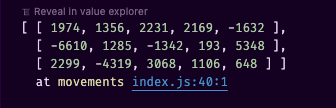# const - JS | lectureWorking With Arrays

flat and flatMap

The next two array methods we'll learn are the `flat` and `flatMap` methods. And thankfully, they are very easy to understand. So let's go.

Let's say we have an array of arrays. That is, an array containing a nested array.

script.js
``const nestedArray = [[1, 2], [3, 4], [5, 6], 7, 8, 9];``

Now the question is, how can we pull out all the elements of the nested array and put them into a single array, which contains all the elements from 1 to 9? Well, that's pretty simple using the `flat` method which was introduced in ES10.

script.js
``````/* ⚠️ NO CALLBACK FUNCTION NEEDED ⚠️ */
console.log(nestedArray.flat()); // [1, 2, 3, 4, 5, 6, 7, 8, 9]``````

But now, let's say we have a more deeply nested array.

script.js
``const deeplyNestedArray = [[[1, 2], [3, 4], [5, 6], 7], [8, [9, 10]], 11, 12];``

Let's see what happens when we use the `flat` method on this array.

script.js
``console.log(deeplyNestedArray.flat()); // [ [ 1, 2 ], [ 3, 4 ], [ 5, 6 ], 7, 8, [ 9, 10 ], 11, 12 ]``

You can see that we get an array which still contains the four inner arrays. This means that, the `flat` method only goes one level deep when flattening the array. For example, `7` was inside the first level of nesting. Hence, it was taken out and it's now in the main array, but then we still have the nested arrays: `[1, 2], [3, 4], [5, 6]`.

We can fix this by using the `depth` argument, which is an integer that specifies how many levels of nesting should be flattened. By default, it's set to 1. So writing `flat(1)` is the same as writing `flat()`.

script.js
``````console.log(deeplyNestedArray.flat(1));

// IS THE SAME AS

console.log(deeplyNestedArray.flat());``````

So, what we need to do is to pass in a value of 2, which means that we want to flatten the array two levels deep.

script.js
``console.log(deeplyNestedArray.flat(2)); // [ 1, 2, 3, 4, 5, 6, 7, 8, 9, 10, 11, 12 ]``

Let's have a more concrete example. Let's consider the below accounts from a bank app.

script.js
``````const accounts = [
{
name: 'Dollie Ingram',
movements: [1974, 1356, 2231, 2169, -1632],
},
{
name: 'Anthony Graham',
movements: [-6610, 1285, -1342, 193, 5348],
},
{
name: 'Christine Harrison',
movements: [2299, -4319, 3068, 1106, 648],
},
];``````

Now, let's say we want to write a function in our app that calculates the overall balance of all the accounts' movements. So, how can we do that 🤔 ? Well, the first thing to do is to take out all the movements from the accounts array and put them into a single array.

script.js
``const movements = accounts.map((acc) => acc.movements);``As you can see, we have an array containing all the movements from all the accounts. We don't want this nested structure. All we want is a single array containing all the movements.

script.js
``````// flat does not need an argument since we only have one level of nesting
const allMovements = movements.flat(); // [ 1974, 1356, 2231, 2169, -1632, -6610, ... ]``````

All that's now left is to calculate the overall balance of all the movements.

script.js
``const overallBalance = allMovements.reduce((acc, curr) => acc + curr, 0); // 7774``

It can be a lot more beautiful using chaining 😌 .

script.js
``````const overallBalance = accounts
.map((acc) => acc.movements)  .flat()  .reduce((acc, curr) => acc + curr, 0); // 7774``````

It turns out that using a map first and then flattening the result is a pretty common operation, which is exactly what we did above. Hence, to solve this, there is another method that was also introduced in ES2019, which is the `flatMap` method.

`flatMap` essentially combines a `map` and a `flat` method into just one method, which is better for performance.

script.js
``````const overallBalance = accounts
.flatMap((acc) => acc.movements)  .reduce((acc, curr) => acc + curr, 0); // 7774``````

The `flatMap` method needs to receive the same callback as the map method since it also does mapping. So it is essentially a map method that flattens the result in the end.

To finish, the `flatMap` method only goes one level deep and we cannot change it. So if you do need to go deeper than just one level, you still need to use the flat method.